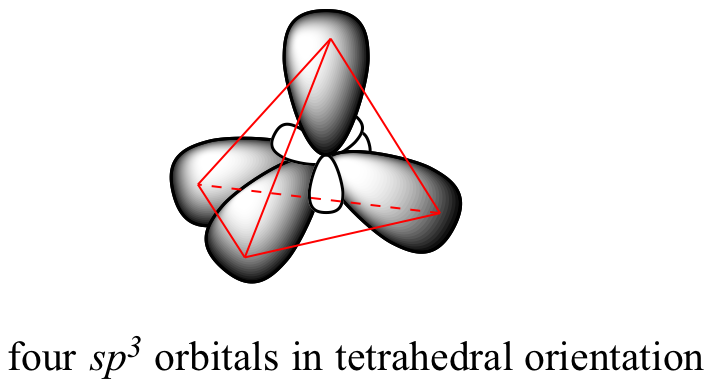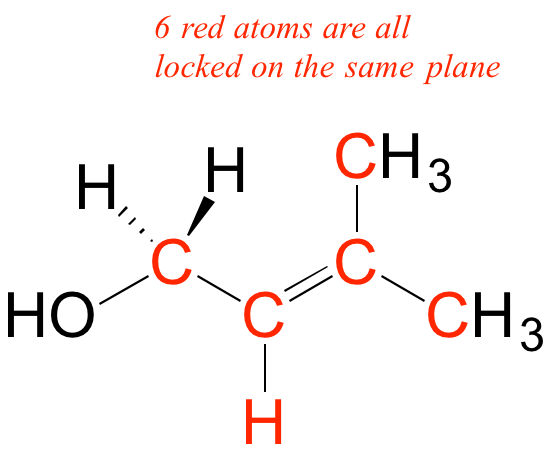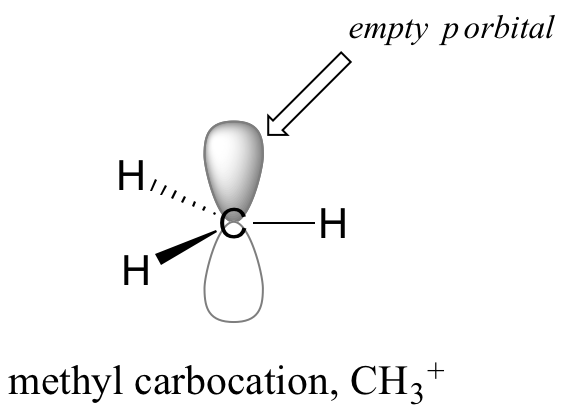Skip to main content

# 2.1: Valence Bond Theory

• Contributed by

As we have been discussing how to use Lewis structures to depict the bonding in organic compounds, we have been very vague so far in our language about the actual nature of the chemical bonds themselves. We know that a covalent bond involves the ‘sharing’ of a pair of electrons between two atoms - but how does this happen, and how does it lead to the formation of a bond holding the two atoms together?

Valence bond theory is most often used to describe bonding in organic molecules. In this model, bonds are considered to form from the overlap of two atomic orbitals on different atoms, each orbital containing a single electron. In looking at simple inorganic molecules such as molecular hydrogen (H2) or hydrogen fluoride (HF), our present understanding of s and p atomic orbitals will suffice. In order to explain the bonding in organic molecules, however, we will need to introduce the concept of hybrid orbitals.

## The sigma bond in the H2 molecule

The simplest case to consider is the hydrogen molecule, H2. When we say that the two hydrogen nuclei share their electrons to form a covalent bond, what we mean in valence bond theory terms is that the two spherical 1s orbitals (the grey spheres in the figure below) overlap, and contain two electrons with opposite spin.These two electrons are now attracted to the positive charge of both of the hydrogen nuclei, with the result that they serve as a sort of ‘chemical glue’ holding the two nuclei together.

How far apart are the two nuclei? If they are too far apart, their respective 1s orbitals cannot overlap, and thus no covalent bond can form - they are still just two separate hydrogen atoms. As they move closer and closer together, orbital overlap begins to occur, and a bond begins to form. This lowers the potential energy of the system, as new, attractive positive-negative electrostatic interactions become possible between the nucleus of one atom and the electron of the second.

But something else is happening at the same time: as the atoms get closer, the repulsive positive-positive interaction between the two nuclei also begins to increase.At first this repulsion is more than offset by the attraction between nuclei and electrons, but at a certain point, as the nuclei get even closer, the repulsive forces begin to overcome the attractive forces, and the potential energy of the system rises quickly. When the two nuclei are ‘too close’, we have an unstable, high-energy situation. There is a defined optimal distance between the nuclei in which the potential energy is at a minimum, meaning that the combined attractive and repulsive forces add up to the greatest overall attractive force. This optimal internuclear distance is the bond length. For the H2 molecule, the distance is 74 pm (picometers, 10-12 meters). Likewise, the difference in potential energy between the lowest energy state (at the optimal internuclear distance) and the state where the two atoms are completely separated is called the bond dissociation energy, or, more simply, bond strength. For the hydrogen molecule, the H-H bond strength is equal to about 435 kJ/mol.

Every covalent bond in a given molecule has a characteristic length and strength. In general, the length of a typical carbon-carbon single bond in an organic molecule is about 150 pm, while carbon-carbon double bonds are about 130 pm, carbon-oxygen double bonds are about 120 pm, and carbon-hydrogen bonds are in the range of 100 to 110 pm. The strength of covalent bonds in organic molecules ranges from about 234 kJ/mol for a carbon-iodine bond (in thyroid hormone, for example), about 410 kJ/mole for a typical carbon-hydrogen bond, and up to over 800 kJ/mole for a carbon-carbon triple bond.

Table of bond lengths and bond energies

It is not accurate, however, to picture covalent bonds as rigid sticks of unchanging length - rather, it is better to picture them as springs which have a defined length when relaxed, but which can be compressed, extended, and bent. This ‘springy’ picture of covalent bonds will become very important in chapter 4, when we study the analytical technique known as infrared (IR) spectroscopy.

One more characteristic of the covalent bond in H2 is important to consider at this point. The two overlapping 1s orbitals can be visualized as two spherical balloons being pressed together. This means that the bond has cylindrical symmetry: if we were to take a cross-sectional plane of the bond at any point, it would form a circle. This type of bond is referred to as a σ (sigma) bond.A sigma bond can be formed by overlap of an s atomic orbital with a p atomic orbital. Hydrogen fluoride (HF) is an example:A sigma bond can also be formed by the overlap of two p orbitals. The covalent bond in molecular fluorine, F2, is a sigma bond formed by the overlap of two half-filled 2p orbitals, one from each fluorine atom.## sp3 hybrid orbitals and tetrahedral bonding

Now let’s look more carefully at bonding in organic molecules, starting with methane, CH4. Recall the valence electron configuration of a carbon atom:This picture is problematic when it comes to describing the bonding in methane. How does the carbon form four bonds if it has only two half-filled p orbitals available for bonding? A hint comes from the experimental observation that the four C-H bonds in methane are arranged with tetrahedral geometry about the central carbon, and that each bond has the same length and strength. In order to explain this observation, valence bond theory relies on a concept called orbital hybridization. In this picture, the four valence orbitals of the carbon (one 2s and three 2p orbitals) combine mathematically (remember: orbitals are described by wave equations) to form four equivalent hybrid orbitals, which are called sp3 orbitals because they are formed from mixing one s and three p orbitals. In the new electron configuration, each of the four valence electrons on the carbon occupies a single sp3 orbital.interactive 3D model
(select 'load sp3' and 'load H 1s' to see orbitals)

This geometric arrangement makes perfect sense if you consider that it is precisely this angle that allows the four orbitals (and the electrons in them) to be as far apart from each other as possible. This is simply a restatement of the Valence Shell Electron Pair Repulsion (VSEPR) theory that you learned in General Chemistry: electron pairs (in orbitals) will arrange themselves in such a way as to remain as far apart as possible, due to negative-negative electrostatic repulsion.

Each C-H bond in methane, then, can be described as a sigma bond formed by overlap between a half-filled 1s orbital in a hydrogen atom and the larger lobe of one of the four half-filled sp3 hybrid orbitals in the central carbon. The length of the carbon-hydrogen bonds in methane is 109 pm.While previously we drew a Lewis structure of methane in two dimensions using lines to denote each covalent bond, we can now draw a more accurate structure in three dimensions, showing the tetrahedral bonding geometry. To do this on a two-dimensional page, though, we need to introduce a new drawing convention: the solid / dashed wedge system. In this convention, a solid wedge simply represents a bond that is meant to be pictured emerging from the plane of the page. A dashed wedge represents a bond that is meant to be pictured pointing into, or behind, the plane of the page. Normal lines imply bonds that lie in the plane of the page. This system takes a little bit of getting used to, but with practice your eye will learn to immediately ‘see’ the third dimension being depicted.

Exercise 2.1: Imagine that you could distinguish between the four hydrogen atoms in a methane molecule, and labeled them Ha through Hd. In the images below, the exact same methane molecule is rotated and flipped in various positions. Draw the missing hydrogen atom labels. (It will be much easier to do this if you make a model.)Exercise 2.2: What kind of orbitals overlap to form the C-Cl bonds in chloroform, CHCl3?

Solutions to exercises

How does this bonding picture extend to compounds containing carbon-carbon bonds? In ethane (CH3CH3), both carbons are sp3-hybridized, meaning that both have four bonds with tetrahedral geometry. The carbon-carbon bond, with a bond length of 154 pm, is formed by overlap of one sp3 orbital from each of the carbons, while the six carbon-hydrogen bonds are formed from overlaps between the remaining sp3 orbitals on the two carbons and the 1s orbitals of hydrogen atoms. All of these are sigma bonds.Because they are formed from the end-on-end overlap of two orbitals, sigma bonds are free to rotate. This means, in the case of ethane molecule, that the two methyl (CH3) groups can be pictured as two wheels on an axle, each one able to rotate with respect to the other.In chapter 3 we will learn more about the implications of rotational freedom in sigma bonds, when we discuss the ‘conformation’ of organic molecules.

The sp3 bonding picture is also used to described the bonding in amines, including ammonia, the simplest amine. Just like the carbon atom in methane, the central nitrogen in ammonia is sp3-hybridized. With nitrogen, however, there are five rather than four valence electrons to account for, meaning that three of the four hybrid orbitals are half-filled and available for bonding, while the fourth is fully occupied by a nonbonding pair (lone pair) of electrons.space-filling image

The bonding arrangement here is also tetrahedral: the three N-H bonds of ammonia can be pictured as forming the base of a trigonal pyramid, with the fourth orbital, containing the lone pair, forming the top of the pyramid. Recall from your study of VSEPR theory in General Chemistry that the lone pair, with its slightly greater repulsive effect, ‘pushes’ the three N-H s bonds away from the top of the pyramid, meaning that the H-N-H bond angles are slightly less than tetrahedral, at 107.3˚ rather than 109.5˚.

VSEPR theory also predicts, accurately, that a water molecule is ‘bent’ at an angle of approximately 104.5˚. The bonding in water results from overlap of two of the four sp3 hybrid orbitals on oxygen with 1s orbitals on the two hydrogen atoms. The two nonbonding electron pairs on oxygen are located in the two remaining sp3 orbitals.Exercise 2.3: Draw, in the same style as the figures above, orbital pictures for the bonding in a) methylamine, and b) ethanol.

Solutions to exercises

video tutorial on sp3 orbitals and sigma bonds

## sp2 and sp hybrid orbitals and pi bonds

The valence bond theory, along with the hybrid orbital concept, does a very good job of describing double-bonded compounds such as ethene. Three experimentally observable characteristics of the ethene molecule need to be accounted for by a bonding model:

1. Ethene is a planar (flat) molecule.
2. Bond angles in ethene are approximately 120o, and the carbon-carbon bond length is 134 pm, significantly shorter than the 154 pm single carbon-carbon bond in ethane.
3. There is a significant barrier to rotation about the carbon-carbon double bond.Clearly, these characteristics are not consistent with an sp3 hybrid bonding picture for the two carbon atoms. Instead, the bonding in ethene is described by a model involving the participation of a different kind of hybrid orbital. Three atomic orbitals on each carbon – the 2s, 2px and 2py orbitals – combine to form three sp2 hybrids, leaving the 2pz orbital unhybridized.The three sp2 hybrids are arranged with trigonal planar geometry, pointing to the three corners of an equilateral triangle, with angles of 120° between them. The unhybridized 2pz orbital is perpendicular to this plane (in the next several figures, sp2 orbitals and the sigma bonds to which they contribute are represented by lines and wedges; only the 2pz orbitals are shown in the 'space-filling' mode).The carbon-carbon double bond in ethene consists of one sigma bond, formed by the overlap of two sp2 orbitals, and a second bond, called a pi bond, which is formed by the side-by-side overlap of the two unhybridized 2pz orbitals from each carbon.animation spacefilling image video tutorial interactive 3D model (select 'show resulting pi orbital')

Unlike a sigma bond, a pi bond does not have cylindrical symmetry. If rotation about this bond were to occur, it would involve disrupting the side-by-side overlap between the two 2pz orbitals that make up the pi bond. The presence of the pi bond thus ‘locks’ the six atoms of ethene into the same plane. This argument extends to larger alkene groups: in each case, six atoms lie in the same plane.Exercise 2.4: Redraw the structures below, indicating the six atoms that lie in the same plane due to the carbon-carbon double bond.Exercise 2.5: What is wrong with the way the following structure is drawn?Solutions to exercises

A similar picture can be drawn for the bonding in carbonyl groups, such as formaldehyde. In this molecule, the carbon is sp2-hybridized, and we will assume that the oxygen atom is also sp2 hybridized. The carbon has three sigma bonds: two are formed by overlap between sp2 orbitals with 1s orbitals from hydrogen atoms, and the third sigma bond is formed by overlap between the remaining carbon sp2 orbital and an sp2 orbital on the oxygen. The two lone pairs on oxygen occupy its other two sp2 orbitals.interactive 3D model

The pi bond is formed by side-by-side overlap of the unhybridized 2pz orbitals on the carbon and the oxygen. Just like in alkenes, the 2pz orbitals that form the pi bond are perpendicular to the plane formed by the sigma bonds.

Exercise 2.6:

a: Draw a diagram of hybrid orbitals in an sp2-hybridized nitrogen.

b: Draw a figure showing the bonding picture for the imine below.c: In your drawing for part b, what kind of orbital holds the nitrogen lone pair?

Solutions to exercises

Recall that carbocations are transient, high-energy species in which a carbon only has three bonds (rather than the usual four) and a positive formal charge. We will have much more to say about carbocations in this and later chapters. For now, though, the important thing to understand is that a carbocation can be described as an sp2-hybridized carbon with an empty p orbital perpendicular to the plane of the sigma bonds.Finally, the hybrid orbital concept applies as well to triple-bonded groups, such as alkynes and nitriles. Consider, for example, the structure of ethyne (common name acetylene), the simplest alkyne.Both the VSEPR theory and experimental evidence tells us that the molecule is linear: all four atoms lie in a straight line. The carbon-carbon triple bond is only 120 pm long, shorter than the double bond in ethene, and is very strong, about 837 kJ/mol. In the hybrid orbital picture of acetylene, both carbons are sp-hybridized. In an sp-hybridized carbon, the 2s orbital combines with the 2px orbital to form two sp hybrid orbitals that are oriented at an angle of 180° with respect to each other (eg. along the x axis). The 2py and 2pz orbitals remain unhybridized, and are oriented perpendicularly along the y and z axes, respectively.The carbon-carbon sigma bond, then, is formed by the overlap of one sp orbital from each of the carbons, while the two carbon-hydrogen sigma bonds are formed by the overlap of the second sp orbital on each carbon with a 1s orbital on a hydrogen. Each carbon atom still has two half-filled 2py and 2pz orbitals, which are perpendicular both to each other and to the line formed by the sigma bonds. These two perpendicular pairs of p orbitals form two pi bonds between the carbons, resulting in a triple bond overall (one sigma bond plus two pi bonds).space-filling image

interactive 3D model

Exercise 2.7: Look at the structure of thiamine diphosphate in the 'structures of common coenzymes' table. Identify the hybridization of all carbon atoms in the molecule.

Solutions to exercises

The hybrid orbital concept nicely explains another experimental observation: single bonds adjacent to double and triple bonds are progressively shorter and stronger than single bonds adjacent to other single bonds. Consider for example, the carbon-carbon single bonds in propane, propene, and propyne.All three are single (sigma) bonds; the bond in propyne is shortest and strongest, while the bond in propane is longest and weakest. The explanation is relatively straightforward. An sp orbital is composed of one s orbital and one p orbital, and thus it has 50% s character and 50% p character. sp2 orbitals, by comparison, have 33% s character and 67% p character, while sp3 orbitals have 25% s character and 75% p character. Because of their spherical shape, 2s orbitals are smaller, and hold electrons closer and ‘tighter’ to the nucleus, compared to 2p orbitals. It follows that electrons in an sp orbital, with its greater s character, are closer to the nucleus than electrons in an sp2 or sp3 orbital. Consequently, bonds involving sp-sp3 overlap (as in propyne) are shorter and stronger than bonds involving sp2-sp3 overlap (as in propene). Bonds involving sp3-sp3 overlap (as in propane) are the longest and weakest of the three.

Exercise 2.8:

a) What kinds of orbitals are overlapping in bonds b-i indicated below? Be sure to distinguish between s and p bonds. An example is provided for bond 'a'.

b) In what kind of orbital is the lone pair of electrons located on the nitrogen atom of bond a? Of bond e?Solutions to exercises

Kahn Academy video tutorial on valence bond theory / hybrid orbitals